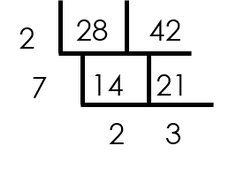For a ‘live’ explanation, check out the video below!

Finding the Least Common Denominator with theIs ‘least common denominator’ your answer to this question: “What’s the most challenging math topic to teach/most difficult for your students to ‘get’?” This question is the one I asked in a recent Instagram survey. I got a variety of responses, but the one that came up most often was fractions – remembering the ‘rules;’ students finding common denominators when they were multiplying; students (older students) not being able to find a common denominator; and so on.

So, today, I’m going to share how to use the ladder method to find the lowest (least) common denominator, and hopefully, if your students have struggled with this, it will help them (and you!). Before I explain how it works, I want to share that I’ve used the ladder method for several years, after many years of teaching GCF and LCM the ‘traditional’ way  – the way I’d been taught! And during those years, I’d often get frustrated by the fact that students would miss the GCF because they missed factors, or they couldn’t find the LCD because the numbers got too big so they just multiplied the denominators…..or they listed out the multiples, but made a mistake in one list, and so they never found an LCM/LCD. I’m sure you know what I mean!

The ladder method took these issues away, and it also added something I didn’t initially expect – it appeared to improve number sense for many students who struggled with their multiplication facts or with the idea of finding factors and multiples. It helped them understand HOW numbers were related to each other by making the breakdown of the #s more visual (using prime factorization does this as well, but the ladder method provides a little more organization to the process, and I think that’s helpful).Finding the LCD
So, here’s how to find LCD with the ladder method.
Let’s use the problem 9/28 + 13/42

Step 1: Put the denominators in the ladder.Step 2: “Remove” common factors.
a)
Choose a common factor of the denominators and place it outside the ladder. The factor can be any factor. I tend to use prime numbers, because we also use the ladder method for prime factorization, and that just keeps things consistent. Students often like to start with small numbers – they are faster and maybe a little ‘safer’.  In this case, I’ll start with 2.b) Divide the numbers in the ladder by the number outside (2) and write the quotients below the original numbers.c) Look at the quotients and determine whether they have a common factor. They can both be divided by 7, so add a 7 outside the ladder and divide again.Check the new quotients for common factors. 2 and 3 have no common factors, so we’re finished with the ladder part.

Step 3: Multiply Factors
Now, to find the LCD (LCM), multiply all the numbers (factors) on the outside of the ladder (2 x 7 x 2 x 3 = 84). The numbers on the left
of the ladder are the factors that the denominators share. The numbers on the bottom are the factors they do not have in common. Multiplying all of them gives us the LCM.

Step 4: Find Equivalent Fractions
So now the common denominator is 84.
Finding equivalent fractions should be a similar process to what students already do – find what 9 and 13 were multiplied by to get 84. Once they go through the process several times, they may notice that the number the numerator needs to be multiplied by is always the factor at the bottom of the ladder, under the OPPOSITE denominator. So, in this case, 9 is multiplied by 3 and 13 is multiplied by 2, to get 27/84 + 26/84.

Once students get the hang of using the ladder method for least common denominator, they can find the LCD very quickly!

I hope this is helpful and I hope you get the chance to use it and see how your students respond!

I created a ‘least common denominator’ notes sheet (free) and a least common denominator math wheel in case you want to share either of them with your students. If you have any questions, please let me know!Are you interested in more fraction and fraction operation content?
Check out the program,Grab this free fractions operations wheel (plus other fraction goodies) when you join the email community!

## EllieWelcome to Cognitive Cardio Math! I’m Ellie, a wife, mom, grandma, and dog ‘mom,’ and I’ve spent just about my whole life in school! With nearly 30 years in education, I’ve taught:

• All subject areas in 4th and 5th grades
• Math, ELA, and science in 6th grade (middle school)

I’ve been creating resources for teachers since 2012 and have worked in the elearning industry for about five years as well!